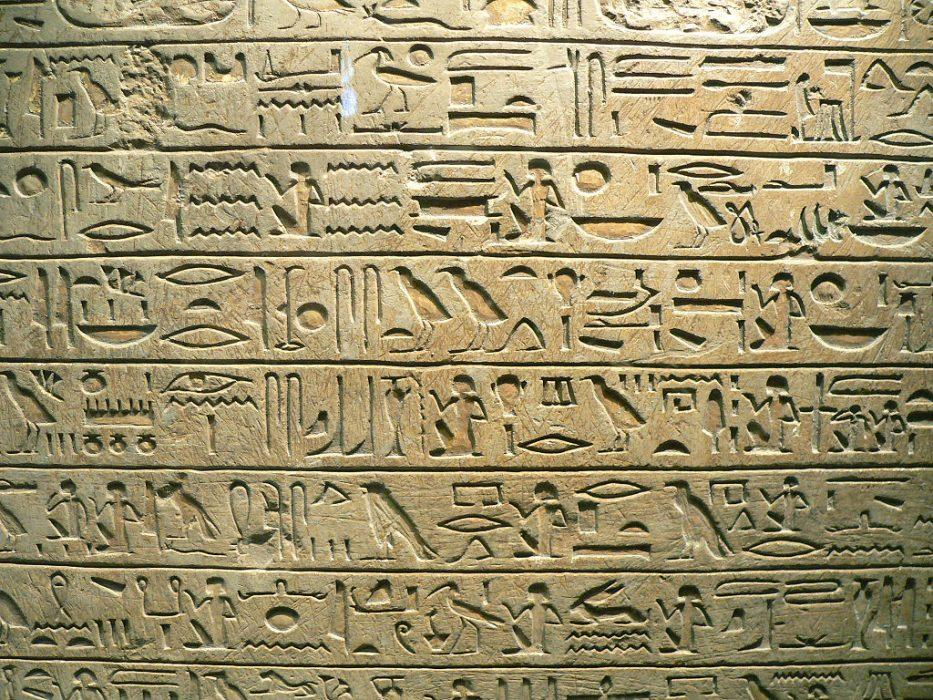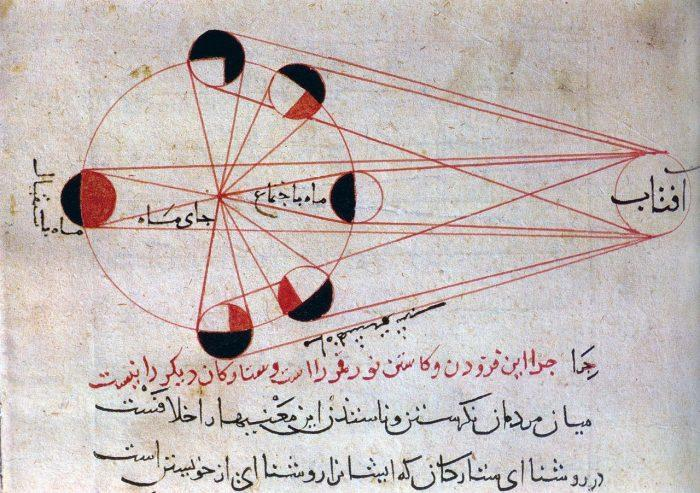Are you curious about maths, from KS2 through to GCSE and degree-level, and what these have to do with Einstein and algebra?

To understand how we got here, we need to follow the long journey from antiquity, from the time of ancient Greek and Arab philosophers. Join us as we trace the spectacular evolution of mathematics through the centuries.

Maths has touched many areas of our society, from computing to art.

We'll find out why the genius of Albert Einstein is inseparable from the history of maths, and delve into the dark corners of maths that they don't teach you about in school!Mansur
Mathematics Teacher4.86 (7) \$30/h
First lesson is free!Abraham
Mathematics Teacher5.00 (4) \$30/h
First lesson is free!Zach
Mathematics Teacher5.00 (9) \$18/h
First lesson is free!Mahshid
Mathematics Teacher5.00 (6) \$30/h
First lesson is free!Safa ur
Mathematics Teacher
\$16/h
First lesson is free!Huakai
Mathematics Teacher
\$15/h
First lesson is free!Abhilash
Mathematics Teacher
\$14/h
First lesson is free!Tai
Mathematics Teacher5.00 (7) \$30/h
First lesson is free!

## The Birth of MathematicsThe ancient Egyptian civilisation is known to have used mathematics (Source: commons.wikimedia.org)

We have to go back all the way back to antiquity to find the origins of maths.

The Egyptians were among the first to have used maths. Objects with clear mathematical uses were unearthed during excavations in the 19th century, demonstrating early civilisations' abilities to solve equations and to conduct complex trade transactions (including the use of mental arithmetic, multiplication, division, calculation, subtraction, addition).

Later, in the time of Plato, Thales and Pythagoras (authors of the famous theorems) arithmetic was invented.

Algebra came about in Alexandria in the 4th century BC.

Elementary mathematics was born with the likes of Euclid, Archimedes of Syracuse and Apollonius of Perge, who gave rise to Euclidean geometry, the study of the circle, statics and the Archimedes' principle. This last discovery made possible for the first time the construction of large boats.

Trigonometry, which describes the relationships between angles and distances in triangles, comes to us thanks to Ptolemy, Pappus and Hipparchus.

For centuries, after these greats minds had faded from memory, maths fell into obscurity, until the golden age of Arabic mathematics, in the 11th century. In the 15th century modern addition, with its + and -, was conceived of by Jean Widmann Edmer. It is the 17th Century, however, when Western maths really entered its golden age:

• Isaac Newton discovers the law of gravity after an apple falls on his head
• René Descartes describes analytical geometry
• Blaise Pascal gives us the calculation of the probability
• Newton gives the world calculusAl-Biruni's explanation of the phases of the moon (Source: wikipedia.org - Al-Biruni)

During the 18th Century, Euler studied functions and Lagrange worked on variations and fluid mechanics, but what has happened during the last two centuries? Advances have been made in number theory, the distribution of prime numbers and more, and the world has enjoyed the fruits of mathematics, including the invention of electricity.

## The Evolution of Maths Teaching

Over the last two centuries, maths learning has changed enormously.

As early as 1818, arithmetic was being taught, free of charge at 'Ragged Schools' set up by John Pounds. From here on, mathematics became increasingly integrated into every child's schooling, so that all who attended school learnt to read, write and count.

In 1833, Parliament voted to allocate funds each year to the construction of schools for poor children, enshrining the concept of basic education, of which maths formed an integral part, as a right for all, in Great Britain.

In 1964, the Schools Council was formed, to regulate, for the first time, the syllabus of exams in the UK.

What about maths education today? Starting in primary school, teachers know that the fundamentals of begin with the development of number fluency.

The use of the calculator at school, too, is a more recent innovation that has become standardised.

But what will maths teaching be like in the future?

## The Essential Mathematics Vocabulary

To study maths, it is important to know the correct vocabulary. To pass a science or maths AS or A2, to pursue a career as a scientist or engineer, to become a researcher or a study for a PhD, you need to at ease with a large lexicon of essential mathematical definitions, including:

• Equations
• Factors
• Products
• Sums
• Terms
• Difference
• Dividend
• Quotient
• Numerator and denominator
• Triangles
• Squares
• Circles

Simple terms like these form the basis of more complex terms, like complex numbers, scalar products, calculus, exponents and absolute values, which form part of a successively more in-depth understanding of maths that make a higher education in the topic possible.

Find the best maths tutor on our platform.Mansur
Mathematics Teacher4.86 (7) \$30/h
First lesson is free!Abraham
Mathematics Teacher5.00 (4) \$30/h
First lesson is free!Zach
Mathematics Teacher5.00 (9) \$18/h
First lesson is free!Mahshid
Mathematics Teacher5.00 (6) \$30/h
First lesson is free!Safa ur
Mathematics Teacher
\$16/h
First lesson is free!Huakai
Mathematics Teacher
\$15/h
First lesson is free!Abhilash
Mathematics Teacher
\$14/h
First lesson is free!Tai
Mathematics Teacher5.00 (7) \$30/h
First lesson is free!

## Maths and Computing

Mathematics and computer science are intimately linked, often by a common vocabulary and logic.

A student acquires a great deal of knowledge in following the National Curriculum and regularly doing maths exercises. But what is to be done with all that knowledge, after completing school? A place at an engineering school or, why not, a career in science, statistics, technology, in teaching maths or a doctorate.

Why not aim for a career in IT? The first computer scientists were themselves mathematicians and some of the most highly paid jobs around today can be found in a domain once reserved for 'geeks'?

Many schools allow you to combine maths and computer science, such as a master's degree in multimedia, business management or data science.

There are diverse jobs related to these two areas, including teacher, engineer, researcher, university lecturer, web developer, programmer, app developer, business manager, graphic designer, even stockbroker.

## Art and Mathematics are Closer Than You Think

The most creative amongst you should remember that maths is not only related to computers.

We can also see very strong links between mathematics and art.

Geometry, for example, is central to drawing and painting. Then there are Thales' and Pythagoras' theorems, which can be seen in symmetry and in colour matching. Drawing and painting, too, are endeavours requiring accuracy and reflection.

We cannot forget to mention the golden ratio in art:

"...the golden ratio is the most mysterious of all compositional strategies. We know that by creating images based on this rectangle our art will be more likely to appeal to the human eye, but we don’t know why."

It can transform even apparently chaotic works into something which is harmonious and pleasant to behold.

Any discussion of maths and art cannot omit to mention the great Leonardo da Vinci. It is to this undisputed genius that we owe the concept of perspective and the Vitruvian Man: a work derived from mathematics.

We also find the golden ratio in his famous works the Mona Lisa and The Last Supper.

## Einstein's Genius

From one mathematical genius to another; perhaps the best-known of them all: He was born in 1879 in Germany and died in Princeton, USA in 1855, Albert Einstein is perhaps the one scientific figure known by all.

There is a popular myth that as a child he was a bad student. This is false: In fact, he was a very good student, but an unruly one. During his university studies, however, he demonstrated a prodigious ability in learning celestial mechanics and nuclear physics.

In 1905 this mathematician and scientist became famous, with the formula E = MC² or the theory of restricted relativity.

The equation states that anything having mass has an equivalent amount of energy and vice versa: The equivalent energy (E) can be calculated as the mass (m) multiplied by the speed of light (c = about 3×108 m/s) squared. Similarly, anything having energy exhibits a corresponding mass (m) given by its energy (E) divided by the speed of light squared (c²).

The theory of general relativity arrived in 1915, drawing on Newton's law of gravity. It explains that what we perceive as the force of gravity arises, in fact, from the curvature of space and time.

Einstein's Mathematical theories have transformed our view of the world, from simplistic notions such as mental arithmetic, multiplication and geometry.

Mathematics is subject to many prejudices and preconceptions.

Read on, as we dispel the following common untruths. Let's start with many people "not getting" maths, for example - a common complaint.

As it turns out, it's a misconception. While certain people do have a special ability for maths, for others, difficulties are most definitely surmountable: No one is condemned to wander in the obscurity of misunderstanding. Private lessons and maths tutors can often come to the rescue.

"Mathematics is useless in real life": Another clearly false statement!

If it were so, how could you calculate the value of a given percentage off the price of an item on sale, for example? Similarly, how could you compare the cheapest flights available for a given route without recourse to the algorithms that give shape to the internet?

"'Maths people' aren't fun" It is quite possible to make learning maths fun, by using many of the readily available tools, games and apps which only grow more numerous and sophisticated every year.

"Art and maths have nothing in common" Geometry is just one example of maths' relevance to art, and one that is used by many of the greats.

"Women are less good at maths than men". Once again - drum roll please - this is false! It's been repeatedly demonstrated that women and men have the same underlying intellectual ability, and it is, instead, society that has traditionally discouraged women from pursuing careers in maths and the sciences.

## Surprising Examples of Maths in Art

Have you heard of 'snow art'?

This is the favoured discipline of Simon Beck, an artist who draws geometric figures in the medium of snow. His portfolio includes over 200 photographs of his gelid creations.

Iranian artist Hamid Naderi Yeganeh works heavily with maths and creates visually arresting motifs, available to view on his website, build on mathematical functions. His work attains a certain harmony through apparently chaotic patterns.

Central among the surprising examples of maths in art, we find fractals. Fractals are mathematical objects whose structure remains constant in spite of any variation in scale.

Maths is also present in 3D mathematical models, mathematical art and 'Fabergé Fractals', and that's just for starters!

Need a teacher Mathematics ?5.00/5, 1 votesLoading...